# GCSE Maths Higher Papers

Here you will find all the free practice higher GCSE mathematics papers and higher maths past papers.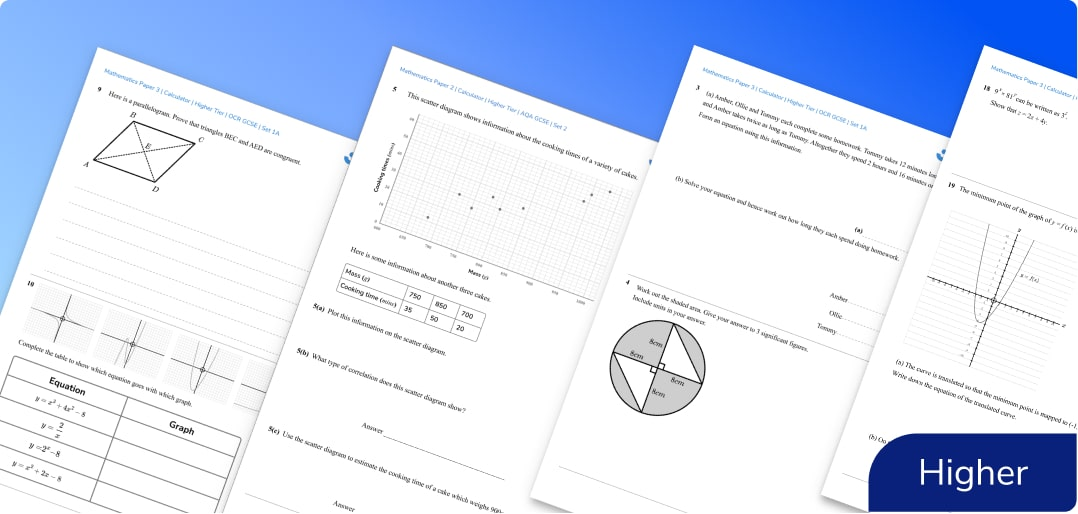What is included in the GCSE maths higher practice papers?

Each set contains three GCSE maths papers: Higher Paper 1 (non-calculator), Higher Paper 2 (calculator), Higher Paper 3 (calculator).

Exam questions are set out in the style of the specimen higher papers from the exam boards and include answers, mark schemes and grade boundaries.

Sets 1a and 1b are based on the higher level maths GCSE advanced information 2022 and past exam papers.

Sets 2 onwards (more to come) are predicted papers based on GCSE maths past papers and the higher tier specification for each board.

## How to use these GCSE maths higher papers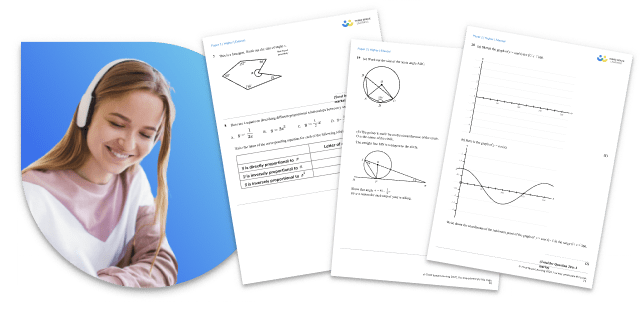These specially created GCSE higher mathematics papers are an essential addition to your GCSE maths revision for the higher tier maths course of most exam boards including WJEC and the IGCSE.

They closely match the past GCSE maths papers and specimen papers provided by the AQA, Edexcel & OCR exam boards. Also useful for WJEC GCSE maths, CCEA or IGCSE maths courses. All maths questions and marking instructions on the exam papers are created by current or former examiners and expert maths teachers.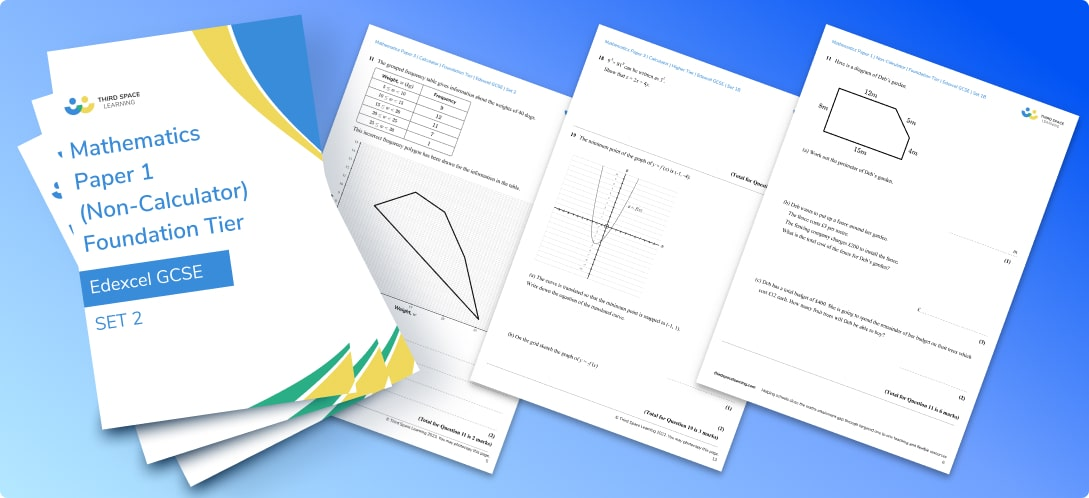While the style and layout of these question papers will be familiar to students, the content is new and unique. Schools use these GCSE higher papers for mock exams as the mark scheme and coverage (including topics like circle theorems, quadratics, vectors, and histograms) enable you to see individual gaps.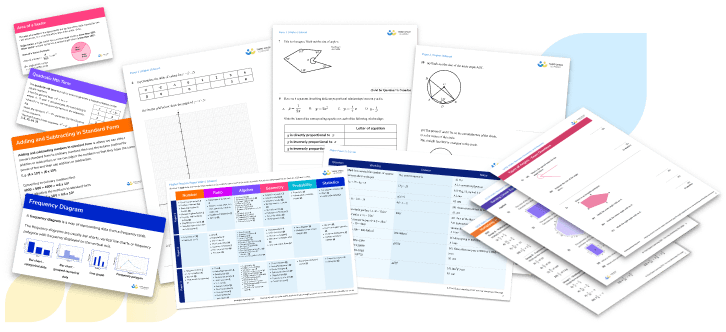More GCSE maths papers

For further GCSE maths exam preparation, take a look at the free worksheets and revision resources below. Also included are free Foundation papers for students aiming for Grades 4 and below.

GCSE Maths Foundation Papers

Edexcel Maths Papers & Past Papers

OCR Maths Papers & Past Papers

## Can't find what you're looking for?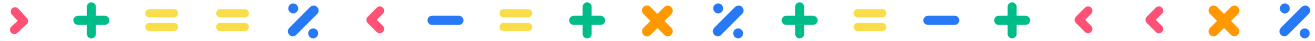## Teaching support from the UK’s largest provider of in-school maths tuition

At Third Space Learning, we’re on a mission to help teachers like you shape your students into confident, able mathematicians.

Request a quote